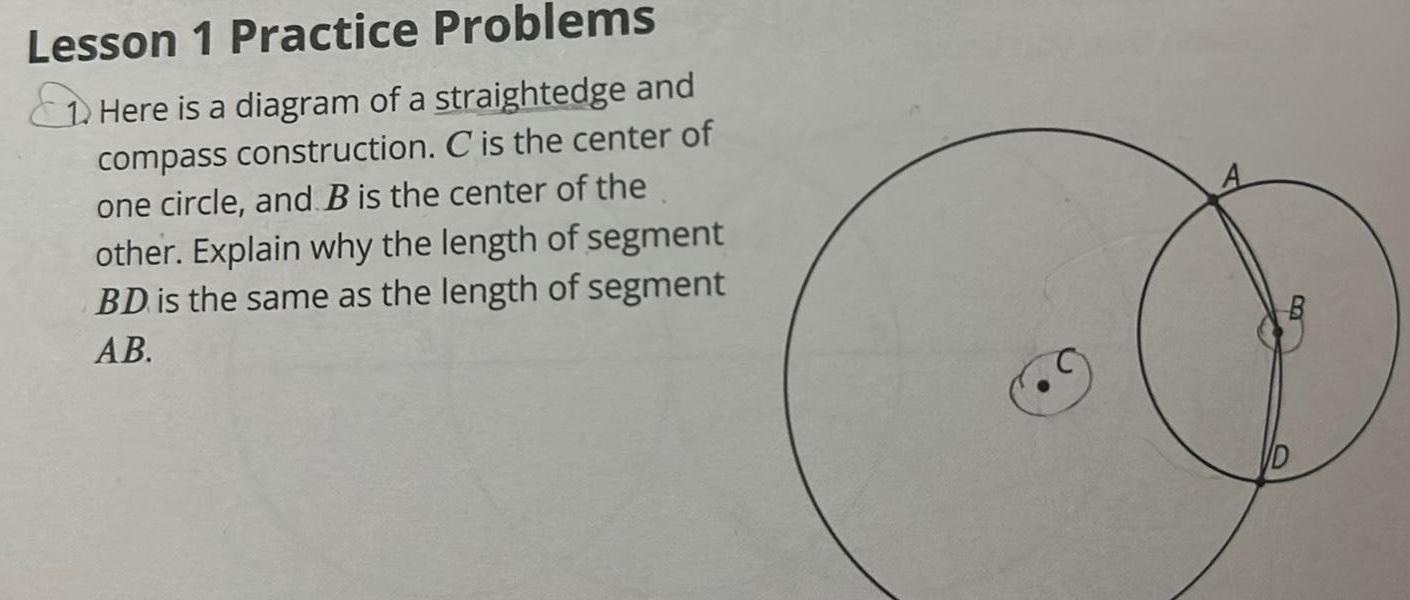Question:

# Lesson 1 Practice Problems Here is a diagram of a

Last updated: 9/12/2023Lesson 1 Practice Problems Here is a diagram of a straightedge and compass construction C is the center of one circle and B is the center of the other Explain why the length of segment BD is the same as the length of segment AB O